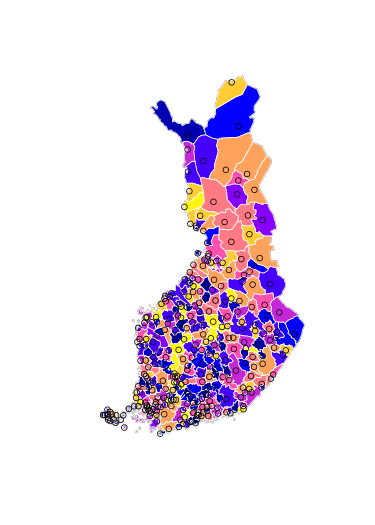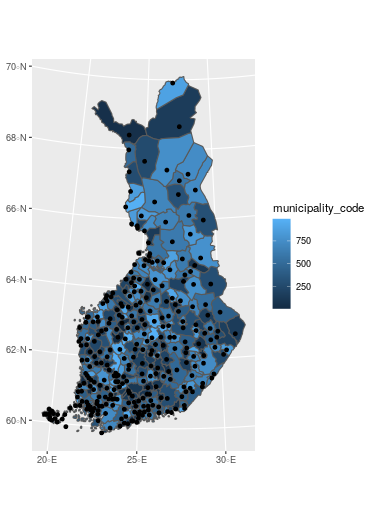# Making maps using geofi-package

#### 2021-03-29

Installation

`geofi` can be installed from CRAN using

``````# install from CRAN
install.packages("geofi")

# Install development version from GitHub
remotes::install_github("ropengov/geofi")``````

This vignettes gives an overview of different options for creating maps in R using the data from `geofi`-package. Vignette is divided in three sections: R-packages for static maps, static maps using ggplot2 and interactive maps. But we begin with the datasets we want to plot.

If you want more detailed explanation of how to plot `sf`-objects take a look at vignette 5. Plotting Simple Features.

## Datasets

Lets start with latest municipality division from `get_municipalities()` with is a POLYGON data and with POINT data of `municipality_central_localities` that is shipped with the package.

``````library(geofi)
polygon <- get_municipalities(year = 2021, scale = 4500)
point <- geofi::municipality_central_localities
# municipality code into integer
point\$municipality_code <- as.integer(point\$kuntatunnus)
library(sf) # for spatial data operations later``````

They both come in same CRS EPSG:3067 and can be plotted together without any further manipulation.

## R-packages for static maps

There are two main technologies for creating static graphics in R: base and ggplot2. Both can be used to plot spatial data ie. to create maps. In addition, tmap : thematic maps in R is a great tool if you want to dig deeper into cartography in R.

base

``````# dev.off()
plot(st_geometry(polygon["municipality_code"]))
plot(st_geometry(point["municipality_code"]), add = TRUE, color = "black")``````ggplot2

``````library(ggplot2)
ggplot() +
geom_sf(data = polygon, aes(fill = municipality_code)) +
geom_sf(data = point)``````tmap

``````library(tmap)
tm_shape(polygon) +
tm_polygons("municipality_code") +
tm_shape(point) +
tm_symbols(col = "black", scale = .5) ``````